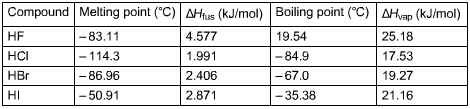Problem: Using the following data, calculate ΔSfus and ΔSvap for HBr.Determine the entropy change when 4.80 mol of HBr (f) freezes at atmospheric pressure.

FREE Expert Solution
87% (245 ratings)
Problem Details

Using the following data, calculate ΔSfus and ΔSvap for HBr.

Determine the entropy change when 4.80 mol of HBr (f) freezes at atmospheric pressure.What scientific concept do you need to know in order to solve this problem?

Our tutors have indicated that to solve this problem you will need to apply the Entropy concept. You can view video lessons to learn Entropy Or if you need more Entropy practice, you can also practice Entropy practice problems .

What is the difficulty of this problem?

Our tutors rated the difficulty of Using the following data, calculate ΔSfus and ΔSvap for HBr.... as medium difficulty.

How long does this problem take to solve?

Our expert Chemistry tutor, Dasha took 8 minutes to solve this problem. You can follow their steps in the video explanation above.

What professor is this problem relevant for?

Based on our data, we think this problem is relevant for Professor Albright's class at UMICH.